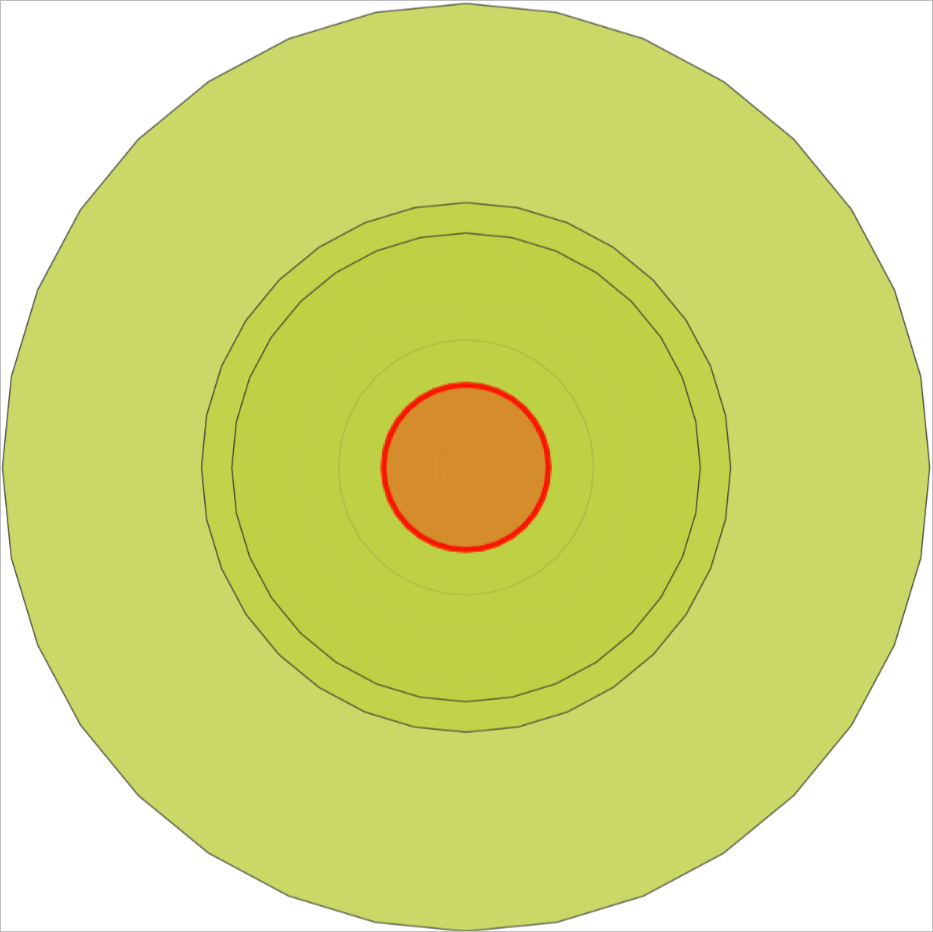This topic describes the ST_Intersection function. This function returns a geometry object corresponding to the set of points where the input geometry objects intersect.

## Syntax

``````geometry  ST_Intersection(geometry  geomA , geometry  geomB);
geography  ST_Intersection(geography  geogA , geography  geogB);
geometry  ST_Intersection(geometry  set gField);``````

## Parameters

Parameter Description
geomA/geomB The two geometry objects that you want to specify.
geogA/geogB The two geography objects that you want to specify.
gField The geometry field that you want to specify.

## Description

• If the two input geometry objects do not intersect, this function returns an empty geometry object.
• If you specify geography objects, this function processes the objects by using geometry functions. This function first converts the input geography objects into geometry objects, calculates the intersection of input objects in the planar spatial reference, and then transforms the intersection to a geography object based on the World Geodetic System 1984 (WGS 84) coordinate system.
• This function does not support GeometryCollection objects.
• This function deletes the m coordinates of the input objects.
• An aggregate function performs an intersection operation on all geometry objects in sequence and returns the intersection of the geometry objects.

## Examples

• Results returned by using the default parameter settings:
``````SELECT ST_AsText(ST_Intersection('POLYGON((0 0,0 2,2 2,2 0,0 0))'::geometry,'POLYGON((0 0,3 0,3 1,0 1,0 0))'::geometry));
st_astext
--------------------------------
POLYGON((0 0,0 1,2 1,2 0,0 0))
(1 row)
``````
• Results returned after an aggregate function is called:
``````create table agg_result(id integer, geom geometry);
insert into agg_result values(0, ST_GeomFromText('POLYGON((0 0, 0 0.5, 0.5 0.5, 0.5 0, 0 0))'));
insert into agg_result select i, st_buffer('POINT(0 0)', 0.8 + random()*0.1) from generate_series(1,100) as i;
select st_astext(st_intersection(geom)) from agg_result;
st_astext
----------------------------------------
POLYGON((0 0,0 0.5,0.5 0.5,0.5 0,0 0))
(1 row)``````